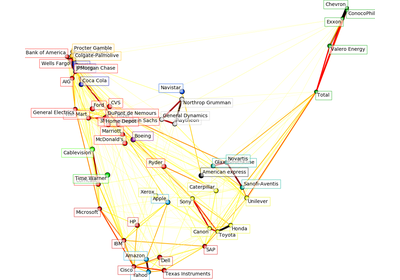# `sklearn.cluster`.affinity_propagation¶

`sklearn.cluster.``affinity_propagation`(S, preference=None, convergence_iter=15, max_iter=200, damping=0.5, copy=True, verbose=False, return_n_iter=False)[source]

Perform Affinity Propagation Clustering of data

Read more in the User Guide.

Parameters: S : array-like, shape (n_samples, n_samples) Matrix of similarities between points preference : array-like, shape (n_samples,) or float, optional Preferences for each point - points with larger values of preferences are more likely to be chosen as exemplars. The number of exemplars, i.e. of clusters, is influenced by the input preferences value. If the preferences are not passed as arguments, they will be set to the median of the input similarities (resulting in a moderate number of clusters). For a smaller amount of clusters, this can be set to the minimum value of the similarities. convergence_iter : int, optional, default: 15 Number of iterations with no change in the number of estimated clusters that stops the convergence. max_iter : int, optional, default: 200 Maximum number of iterations damping : float, optional, default: 0.5 Damping factor between 0.5 and 1. copy : boolean, optional, default: True If copy is False, the affinity matrix is modified inplace by the algorithm, for memory efficiency verbose : boolean, optional, default: False The verbosity level return_n_iter : bool, default False Whether or not to return the number of iterations. cluster_centers_indices : array, shape (n_clusters,) index of clusters centers labels : array, shape (n_samples,) cluster labels for each point n_iter : int number of iterations run. Returned only if return_n_iter is set to True.

Notes

See examples/cluster/plot_affinity_propagation.py for an example.

References

Brendan J. Frey and Delbert Dueck, “Clustering by Passing Messages Between Data Points”, Science Feb. 2007

## Examples using `sklearn.cluster.affinity_propagation`¶Visualizing the stock market structure